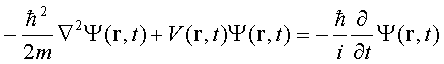SEARCH HOMEMath Central Quandaries & QueriesQuestion from Kara, a student: Do you use complex numbers in your job?Kara, we have 2 responses for you:From Sue:Hi Kara.

I am studying physics and complex and imaginary numbers are extremely important and used in our daily calculations. Here is a clipping from the web which puts it well:

 Not only are complex numbers extremely useful in the subject of mathematics, they play an essential role in many areas of Physics - Electromagnetic Theory, Electronics, AC Circuit Theory, Atomic & Nuclear Physics, Quantum Physics, Wave Theory and Signal Analysis just to name a few.  In many situations in Physics, the real solutions to a problem would be inaccessible without the use of complex numbers.  For instance, Schrödinger's Equation, the basic equation of wave mechanics expressing the behaviour of a particle moving in a field of force, contains the imaginary number i (see below).Stephen La Rocque.>From Penny:Hi Kara,

I am a mathematician and mathematicians use complex numbers very often. They do so in their abstract mathematical work but also in the application of mathematics to practical situations. Here is one example.

If you suspend a coil spring with a weight at the end of the spring, pull the weight down and then release it the weight will oscillate up and down. You can find a simulation at Physics Java Applets, just pull down the weight and release it. To construct a mathematical expression for this motion you can use some knowledge of the physics and calculus. A key step in this procedure is the solution of a quadratic equation which has complex solutions. It is exactly these complex solutions that give rise to the periodic functions that describe the oscillations.

PennyMath Central is supported by the University of Regina and The Pacific Institute for the Mathematical Sciences.